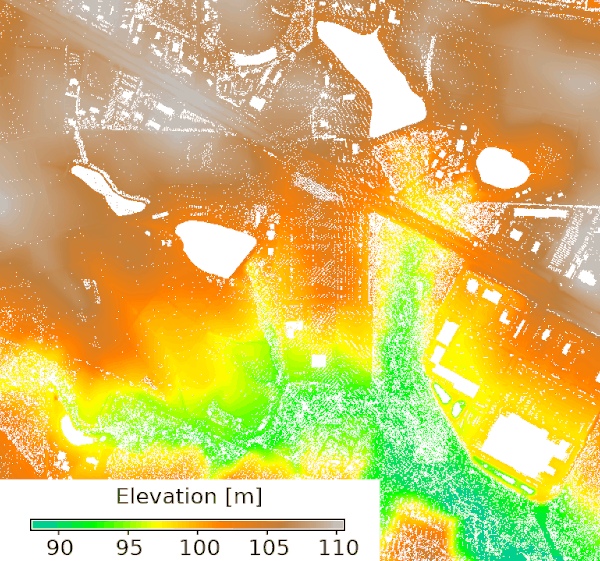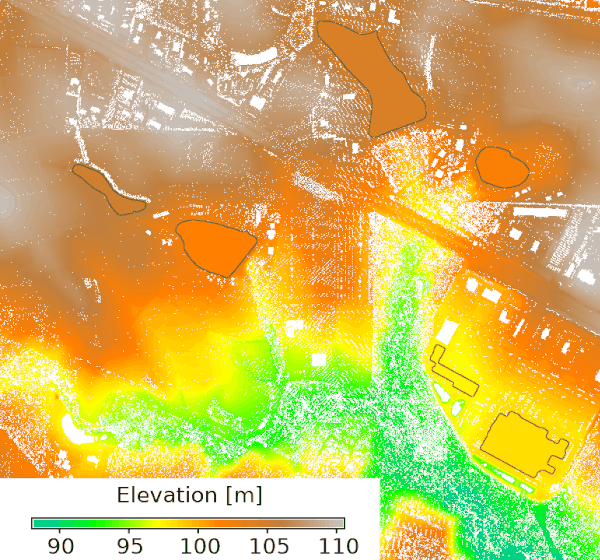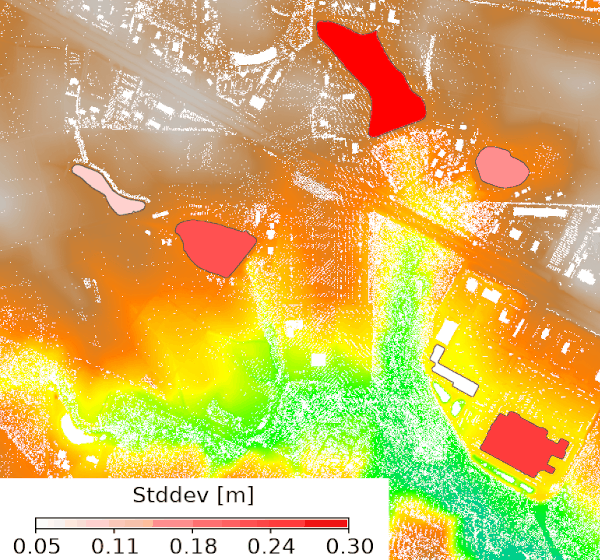## NAME

r.hydro.flatten - Derive elevation of water bodies for hydro-flattening

## KEYWORDS

raster, elevation, hydrology, lidar, LIDAR

## SYNOPSIS

r.hydro.flatten
r.hydro.flatten --help
r.hydro.flatten [-k] input=name water_elevation=name water_elevation_stddev=name percentile=float [min_size=integer] [--overwrite] [--help] [--verbose] [--quiet] [--ui]

### Flags:

-k
Keep intermediate results
--overwrite
Allow output files to overwrite existing files
--help
Print usage summary
--verbose
Verbose module output
--quiet
Quiet module output
--ui
Force launching GUI dialog

### Parameters:

input=name [required]
Raster map of binned lidar point elevation
water_elevation=name [required]
Raster map of derived water elevation
Represents single elevation value for each water body
water_elevation_stddev=name [required]
Raster map of derived water elevation standard deviation
percentile=float [required]
Percentile of elevation to determine water level
Default: 5
min_size=integer
Minimum size of areas in map units

## DESCRIPTION

The tool derives single elevation value for water bodies based on lidar data. These values are used for hydro-flattening a digital elevation model. The input raster is expected to represent ground surface created by binning lidar data (e.g., using r.in.pdal) with averaged ground elevation. Small gaps in the input are expected. Large gaps are interpreted as water bodies. The minimum size of a water body can be set with min_size option in map units.

The output water_elevation is a raster map of water bodies where each water body has a single value representing the water level elevation derived from the lidar data at the edge of a water body. Since the elevation varies along the edge, option percentile is used to determine a single value. The variation along the edge can be examined with the water_elevation_stddev output representing the standard deviation of the lidar elevation values along the water body's edge. Higher deviation suggests problematic areas that need to be further inspected.

To keep the intermediate results for inspection, use flag -k.

## NOTES

While this tool was designed for water bodies, it can be used for other purposes, e.g., for filling a gap in digital elevation models caused by excluding buildings.

This tool does not interpolate gaps in data, rather it derives a single value for each gap. The result can be used to fill gaps and the tool can be run on large areas. For actual gap interpolation, which is typically more computationally intensive, see r.fillnulls.

## EXAMPLE

We will download a lidar tile with r.in.usgs addon, use r.in.pdal to bin the elevation points at 1 meter resolution, and derive elevation levels for lakes with minimum size of 4000 m^2.
```# select study area and resolution
g.region n=213300 s=211900 w=653900 e=655300 res=1
r.in.usgs product=lidar output_directory=/tmp title_filter=Phase2 -d
# bin point elevation using ground and road points with reprojection
r.in.pdal input=/tmp/USGS_LPC_NC_Phase2_2014_LA_37_20164902_.laz output=ground -w class_filter=2,13
# convert elevation from feet to meters
r.mapcalc "ground_m = ground * 0.304800609601219"
# derive elevation of water bodies and standard deviation
r.hydro.flatten input=ground_m water_elevation=water_elevation water_elevation_stddev=water_elevation_stddev percentile=10 misize=4000
```Figure: Input binned elevation representing ground with gaps (left), input overlayed with elevation values estimated for gaps and highlighted with an outline (middle), input overlayed with standard deviation of the elevation along the edge of the gaps (right).

## REFERENCE

Method based on workflow presented at NC GIS Conference 2021 by Doug Newcomb.

r.in.pdal, r.in.usgs, r.fillnulls

## AUTHOR

Anna Petrasova, NCSU GeoForAll Lab

## SOURCE CODE

Available at: r.hydro.flatten source code (history)